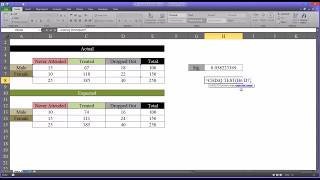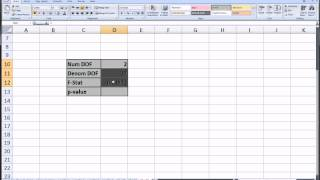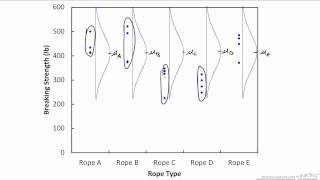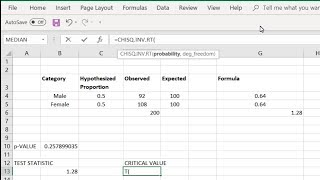Excel Tips & Tutorials:
Chi-Square and Analysis of Variance
 Suggest a Link Alphabetize Page Printer-Friendly List
 More Excel Tips & Tutorials: Select Page ... Getting Started with Excel Data Analysis with Excel Descriptive Statistics Creating and Transforming Variables Making Comparisons Graphing Relationships and Describing Patterns Random Assignment and Sampling Making Controlled Comparisons Foundations of Statistical Inference Hypothesis Tests with One and Two Samples Chi-Square and Analysis of Variance Correlation and Bivariate Regression Multiple Regression Analyzing Regression Residuals Logistic Regression Doing Your Own Political Analysis Advanced Political Analysis with Excel
Watch and Learn
Watch and Learn
Watch and Learn
Watch and Learn
 How to Conduct a Chi-Square TestFrom Todd Grande (8:03).How to Calculate P-Value for an F-StatisticUses Excel F.DIST function (2:26)One-Way ANOVA with the Data Analysis ToolPakNice illustration of underlying data, from Jeffrey Knutsen (4:19)How to Use CHISQ.TEST and CHISQ.INV.RT FunctionsIllustrates with a goodness of fit test (4:12)How to Conduct a Chi-Square TestFrom Todd Grande (8:03).How to Calculate P-Value for an F-StatisticUses Excel F.DIST function (2:26)Calculating Lambda Using Excel Based on given cross-tabulation (8:48)Multiple Applications of ANOVA Real Statistics Using Excel provides detailed instructions for implementing different types of ANOVA with Excel.Chi-Square and F Distributions Excellent references for Chi-Square Test and ANOVA featuring Excel.Calculating Lambda Using Excel Based on given cross-tabulation (8:48)Multiple Applications of ANOVA Real Statistics Using Excel provides detailed instructions for implementing different types of ANOVA with Excel.